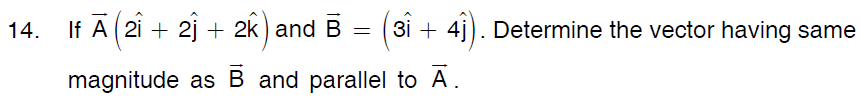# Class-11 Physics (Sample Papers For Practice)### 11. In the given system of masses m1= 5 kg, and coefficient of friction for each constant is 0.2. Calculate the mass m2, if m1 is sliding down with an acceleration of 2 ms–2. What will be the tension in the string?### 13. A bomb is exploded into three fragments of mass 1:2:3. The fragment having lighter masses move with a speed of 40 m/s in mutually perpendicular to each other. Calculate the velocity of the third fragment.### 30. A projectile is fired in air making an angle θ with horizontal. Show that(i) Its path is parabolic in nature.(ii) Tan θ = 4H/R where H is maximum height attained and R is the range of projectile..

Announcements

Join our Online JEE Test Series for 499/- Only (Web + App) for 1 Year

Join our Online NEET Test Series for 499/- Only for 1 Year

1.